# US8779701B2 - Control apparatus for permanent magnet motor - Google Patents

## Info

Publication number
US8779701B2
US8779701B2 US13/029,742 US201113029742A US8779701B2 US 8779701 B2 US8779701 B2 US 8779701B2 US 201113029742 A US201113029742 A US 201113029742A US 8779701 B2 US8779701 B2 US 8779701B2
Authority
US
United States
Prior art keywords
axis
magnet motor
torque
permanent magnet
value
Prior art date
Legal status (The legal status is an assumption and is not a legal conclusion. Google has not performed a legal analysis and makes no representation as to the accuracy of the status listed.)
Active, expires
Application number
US13/029,742
Other versions
US20110241586A1 (en
Inventor
Kazuaki Tobari
Rikio Yoshikawa
Katsuhiro Mochizuki
Current Assignee (The listed assignees may be inaccurate. Google has not performed a legal analysis and makes no representation or warranty as to the accuracy of the list.)
Kokusan Denki Co Ltd
Original Assignee
Kokusan Denki Co Ltd
Priority date (The priority date is an assumption and is not a legal conclusion. Google has not performed a legal analysis and makes no representation as to the accuracy of the date listed.)
Filing date
Publication date
Priority to JP2010-076564 priority Critical
Priority to JP2010076564A priority patent/JP2011211815A/en
Application filed by Kokusan Denki Co Ltd filed Critical Kokusan Denki Co Ltd
Assigned to KOKUSAN DENKI CO., LTD. reassignment KOKUSAN DENKI CO., LTD. ASSIGNMENT OF ASSIGNORS INTEREST (SEE DOCUMENT FOR DETAILS). Assignors: MOCHIZUKI, KATSUHIRO, YOSHIKAWA, RIKIO, TOBARI, KAZUAKI
Publication of US20110241586A1 publication Critical patent/US20110241586A1/en
Application granted granted Critical
Publication of US8779701B2 publication Critical patent/US8779701B2/en
Active legal-status Critical Current

• 238000001514 detection method Methods 0.000 claims description 35
• 230000001276 controlling effect Effects 0.000 claims description 31
• 229910052802 copper Inorganic materials 0.000 claims description 2
• 239000010949 copper Substances 0.000 claims description 2
• 230000001629 suppression Effects 0.000 description 32
• 238000006243 chemical reaction Methods 0.000 description 12
• 230000007274 generation of a signal involved in cell-cell signaling Effects 0.000 description 11
• 238000010586 diagram Methods 0.000 description 9
• 239000000470 constituent Substances 0.000 description 3
• 230000000875 corresponding Effects 0.000 description 2
• 230000000694 effects Effects 0.000 description 2
• 230000004907 flux Effects 0.000 description 2
• 239000003638 reducing agent Substances 0.000 description 2
• 229920002574 CR-39 Polymers 0.000 description 1
• 235000020127 ayran Nutrition 0.000 description 1
• 230000001808 coupling Effects 0.000 description 1
• 238000010168 coupling process Methods 0.000 description 1
• 238000005859 coupling reaction Methods 0.000 description 1
• 238000000034 method Methods 0.000 description 1
• 230000004048 modification Effects 0.000 description 1
• 238000006011 modification reaction Methods 0.000 description 1

## Images

•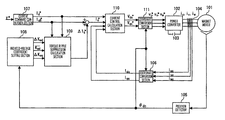•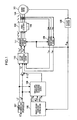•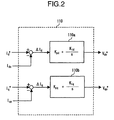•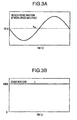•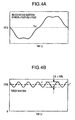•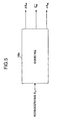•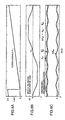•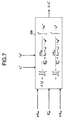•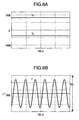•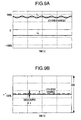•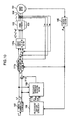•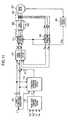•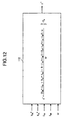•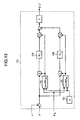•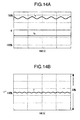•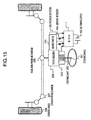## Classifications

• HELECTRICITY
• H02GENERATION; CONVERSION OR DISTRIBUTION OF ELECTRIC POWER
• H02PCONTROL OR REGULATION OF ELECTRIC MOTORS, ELECTRIC GENERATORS OR DYNAMO-ELECTRIC CONVERTERS; CONTROLLING TRANSFORMERS, REACTORS OR CHOKE COILS
• H02P21/00Arrangements or methods for the control of electric machines by vector control, e.g. by control of field orientation
• H02P21/06Rotor flux based control involving the use of rotor position or rotor speed sensors
• HELECTRICITY
• H02GENERATION; CONVERSION OR DISTRIBUTION OF ELECTRIC POWER
• H02PCONTROL OR REGULATION OF ELECTRIC MOTORS, ELECTRIC GENERATORS OR DYNAMO-ELECTRIC CONVERTERS; CONTROLLING TRANSFORMERS, REACTORS OR CHOKE COILS
• H02P21/00Arrangements or methods for the control of electric machines by vector control, e.g. by control of field orientation
• H02P21/22Current control, e.g. using a current control loop

## Abstract

A sinusoidal signal is superimposed on a current command value of a q-axis (torque shaft) supplied from a host, and according to the resulting current command value, the output voltage of a power converter is controlled. For the calculation of the superimposed signal added to the current command value of the q-axis, with the use of the ripple component information of induced voltage coefficients of the d-axis and q-axis of the rotating coordinate system of a permanent magnet motor, the current command values of the d-axis and q-axis, an average value of induced voltage coefficients of the d-axis, and inductance values of the d-axis and q-axis, the sinusoidal superimposed signal is calculated and added to the above-described current command value of the q-axis.

## Description

BACKGROUND OF THE INVENTION
The present invention relates to a torque control apparatus for a permanent magnet motor, and in particular relates to the control apparatus for a permanent magnet motor capable of suppressing a torque ripple when there is a reluctance torque and there is an induced voltage distortion in an induced voltage waveform and thereby realizing an accurate torque control.
As the method of suppressing a torque ripple when the induced voltage of a magnet motor has a square waveform (the induced voltage distortion), there is known a method comprising the steps of converting a three-phase induced voltage waveform into a voltage ed of a d-axis and a voltage eq of a q-axis and calculating a current command value Iqref of the q-axis (canceling the torque ripple) according to Formula (1), as in the description of JPA-2004-201487.
$I qref = 2 3 ⁢ ( T ref × ω m e q ) ( 1 )$
Where
• Tref: a torque command value, and
• ωm: the mechanical angle velocity of a motor.
That is, even if the induced voltage waveform of a magnet motor is a square waveform, the current command value Iqref of the q-axis containing a ripple waveform is calculated so as to set the output torque to be constant.
If vector control is performed according to this current command value, the torque ripple can be suppressed.
Formula (2) is the output torque formula of a magnet motor.
$τ m = 3 2 · P m · [ K ed · I q - K eq · I d + ( L d - L q ) ⁢ I d · I q ] ( 2 )$
Where
• Pm: the number of pole pairs of a motor,
• Ked: the induced voltage coefficient of the d-axis,
• Keq: the induced voltage coefficient of the q-axis,
• Id: the current of the d-axis,
• Iq: the current of the q-axis,
• Ld: the inductance value of the d-axis, and
• Lq: the inductance value of the q-axis.
The method described in JP-A-2004-201487 is a technique corresponding to a non-salient pole type (Ld=Lq) magnet motor with no reluctance torque, wherein the “induced voltage coefficients Ked, Keq” are distorted. Therefore, this method cannot be directly applied to the salient pole type (Ld<Lq) magnet motor.
SUMMARY OF THE INVENTION
In the method described in JP-A-2004-201487, the control is performed so as to cancel the torque ripple component which is generated when the induced voltage waveform is a square waveform. With regard to the output torque, the above method comprises the step of controlling so as to set the component (Ked●Iq−Keq●Id) in Formula (2) to zero, but does not take into consideration the case where there is the (Ld−Lq) Id●Iq component.
In contrast, the purpose of the present invention is to provide a control apparatus for a magnet motor capable of arbitrarily controlling in a predetermined relationship the “ripple component” and “DC component” of the torque ripple in the salient pole type (Ld<Lq) magnet motor.
In order to solve the above-described problems, according to a first aspect of the present invention, a control apparatus for a permanent magnet motor, comprising a power converter which drives the permanent magnet motor and controlling an output of the power converter, performs the steps of calculating a sinusoidal superimposed signal with the use of an induced voltage coefficient of the permanent magnet motor and information about an inductance; adding the superimposed signal to a current command value and controlling the output voltage of the power converter; and controlling the values of a DC torque component and ripple torque component of the permanent magnet motor.
Moreover, in order to solve the above-described problems, according to a second aspect of the present invention, a control apparatus for a permanent magnet motor, comprising a power converter which drives the permanent magnet motor and controlling an output of the power converter, performs the steps of: calculating a sinusoidal superimposed signal with the use of ripple components of an induced voltage coefficient of a d-axis and q-axis of the permanent magnet motor, inductances of the d-axis and q-axis, and current command values of the d-axis and q-axis; adding the superimposed signal to the current command value of the q-axis and controlling the output voltage of the power converter; and controlling the values of a DC torque component and ripple torque component of the permanent magnet motor.
Moreover, in the control apparatus for a permanent magnet motor of the second aspect of the present invention, the calculation of the sinusoidal superimposed signal is performed using a ripple component of an induced voltage coefficient of at least one of the d-axis and q-axis of a rotating coordinate system, a current command value of the other axis, an average value of induced voltage coefficients of the d-axis, inductance values of the d-axis and q-axis, and a current command value of the d-axis.
Moreover, in the control apparatus for a permanent magnet motor of the second aspect of the present invention, the superimposed signal added to the current command value of the q-axis is obtained by setting an arbitrary n in the following calculation formula.
$Δ ⁢ ⁢ I q * = ∑ n = 1 ∞ ⁢ ( - Δ ⁢ ⁢ K ed K ed _ + ( L d - L q ) · I d ⁢ ⁢ 0 * ) n · I q ⁢ ⁢ 0 * - ∑ n = 1 ∞ ⁢ ( - Δ ⁢ ⁢ K eq K ed _ + ( L d - L q ) · I d ⁢ ⁢ 0 * ) n · I d ⁢ ⁢ 0 *$
Where
• n: an integer,
• ΔKed: the “ripple component” of the induced voltage coefficient of the d-axis,
• ΔKeq: the “ripple component” of the induced voltage coefficient of the q-axis,
• Ked : the “DC component” of the induced voltage coefficient of the d-axis,
• Id0*: a torque command value or a current command value of the d-axis supplied from a host, and
• Iq0*: a torque command value or a current command value of the q-axis supplied from the host
Furthermore, in the control apparatus for a permanent magnet motor of the second aspect of the present invention, the ripple component is a signal which varies with a motor position.
Moreover, in order to solve the above-described problems, according to a third aspect of the present invention, a control apparatus for a permanent magnet motor, comprising a power converter which drives the permanent magnet motor and controlling an output of the power converter, performs the steps of calculating a sinusoidal superimposed signal with the use of information about an induced voltage coefficient of a stator coordinate system of the permanent magnet motor and an inductance value; adding the superimposed signal to a three-phase current command value of the stator coordinate system and controlling the output voltage of the power converter; and controlling the values of a DC torque component and ripple torque component of the permanent magnet motor.
Moreover, in order to solve the above-described problems, according to a fourth aspect of the present invention, a control apparatus for a permanent magnet motor, comprising a power converter which drives the permanent magnet motor and controlling an output of the power converter, performs the steps of: estimating the output torque of the permanent magnet motor with the use of information about a voltage and current of the power converter which drives the permanent magnet motor; adding a sinusoidal superimposed signal, which is calculated using a deviation from the torque command value supplied from the host, to the current command value of the q-axis; controlling the output voltage of the power converter; and controlling the values of a DC torque component and ripple torque component of the permanent magnet motor.
Furthermore, in the control apparatus for a permanent magnet motor according to the fourth aspect of the present invention, the method of estimating the output torque of the permanent magnet motor comprises the step of subtracting a copper loss component and a transitional component from effective power information of the power converter.
Furthermore, in the control apparatus for a permanent magnet motor according to the fourth aspect of the present invention, the output torque estimation of the permanent magnet motor is performed according to the following calculation formula.
$τ ⋀ = ( V dc * · I dc + V qc * · I qc ) - R · ( I dc 2 + I qc 2 ) - ( L d · I dc 2 + L q · I qc 2 ) · s ω · 3 2 · P m$
Where
• n: an integer,
• Vdc*: a voltage command value of the d-axis,
• Idc: a current detection value of the d-axis,
• Vqc*: a voltage command value of the q-axis,
• Iqc: a current detection value of the q-axis,
• Ld: an inductance value of the d-axis,
• Lq: an inductance value of the q-axis,
• ω: an electrical angular velocity of the magnet motor,
• Pm: the number of pole pairs, and
• s: Laplace operator.
Furthermore, in the control apparatus for a permanent magnet motor according to the fourth aspect of the present invention, the calculation of the sinusoidal superimposed signal added to the current command value of the q-axis comprises the steps of: calculating a torque deviation between an estimated output torque of the permanent magnet motor and a torque command value supplied from a host, preparing a sine (sin) signal and a cosine (cos) signal with the use of the largest each frequency component contained in a torque estimation value in one period of electric angle of a position detection value, performing a proportional/integral calculation with the use of the cosine (cos) signal and the torque deviation (or the torque estimation value); multiplying the resulting calculation value again by the cosine (cos) signal (this calculation result is referred to as a first calculation result), performing a proportional/integral calculation with the use of the sine (sin) signal and the torque deviation (or the torque estimation value); multiplying the resulting calculation value again by the sine (sin) signal (this calculation result is referred to as a second calculation result); and adding a value, which is obtained by doubling an added value of the first calculation result and the second calculation result, to the current command value of the q-axis supplied from the host.
Furthermore, the control apparatus for a permanent magnet motor according to the fourth aspect of the present invention performs the steps of setting a plurality of angular frequency components contained in the torque estimation value; preparing a plurality of sine (sin) signals and cosine (cos) signals; performing a proportional/integral calculation with the use of the plurality of cosine (cos) signals and torque deviations (or torque estimation values), respectively; multiplying each resulting calculation value again by the plurality of cosine (cos) signals (this calculation result is referred to as a third calculation result); performing a proportional/integral calculation with the use of the plurality of sine (sin) signals and the torque deviations (or the torque estimation value), respectively; multiplying the resulting calculation value again by the plurality of sine (sin) signals (this calculation result is referred to as a fourth calculation result); and adding a value, which is obtained by doubling an added value of the third calculation result and the fourth calculation result, to the current command value of the q-axis supplied from the host.
Furthermore, the control apparatus for a permanent magnet motor according to the first aspect of the present invention performs, as the method of suppressing a torque ripple, the method of suppressing a torque ripple of the second aspect of the present invention.
Furthermore, according to a fifth aspect of the present invention, an electric power steering control apparatus includes the control apparatus for a permanent magnet motor according to the first aspect of the present invention.
Furthermore, in the control apparatus for a permanent magnet motor according to the first aspect of the present invention, for the ripple frequency of a torque ripple, the DC torque component increases by a magnitude of a half the ripple torque component of the 12th order harmonic component.
Furthermore, in the control apparatus for a permanent magnet motor according to the first aspect of the present invention, by arbitrarily setting a magnitude of the current value of the d-axis, the “ripple torque component of the 12th order harmonic component” and the “DC torque component which increases more than the torque command value” have a relationship as expressed by the following formulas.
Ripple Torque Component:
$Δ ⁢ ⁢ τ m ≈ 3 2 · P m · [ 1 2 · ( cos ⁡ [ 12 ⁢ ⁢ θ ] - sin ⁡ [ 12 ⁢ ⁢ θ ] ) · I d _ · K ed 2 _ K ed _ + ( L d - L q ) · I d _ ]$
DC Torque Component:
$Δ ⁢ ⁢ τ m _ ≈ 3 2 · P m · [ - 1 2 · I d _ · K ed 2 _ K ed _ + ( L d - L q ) · I d _ ]$
According to the present invention, in a control apparatus for a permanent magnet motor, the torque ripple of the magnet motor wherein there is a reluctance torque is suppressed. Moreover, the “ripple component” and “DC component” of the torque ripple can be arbitrarily controlled in a predetermined relationship, and accurate torque control characteristics can be realized.
Other objects, features and advantages of the invention will become apparent from the following description of the embodiments of the invention taken in conjunction with the accompanying drawings.
BRIEF DESCRIPTION OF THE DRAWINGS
FIG. 1 illustrates a block diagram of a control apparatus for a permanent magnet motor according to one embodiment of the present invention.
FIG. 2 illustrates a block diagram of a current control calculation section.
FIGS. 3A and 3B illustrate a torque characteristic when an induced voltage waveform of a magnet motor is a sine waveform.
FIGS. 4A and 4B illustrate a torque characteristic when the induced voltage waveform of the magnet motor is distorted.
FIG. 5 illustrates a block diagram of an induced-voltage coefficient setting section which is a feature of the present invention.
FIGS. 6A-6C illustrate a relational diagram of a position signal and an information signal of an induced voltage coefficient.
FIG. 7 illustrates a block diagram of a torque ripple suppression control calculation section which is a feature of the present invention.
FIGS. 8A and 8B illustrate the torque control characteristics when a magnet motor whose induced voltage waveform is distorted is sinusoidally driven.
FIGS. 9A and 9B illustrate the torque control characteristics when the present invention is used.
FIG. 10 illustrates another embodiment of the present invention.
FIG. 11 illustrates yet another embodiment of the present invention.
FIG. 12 illustrates a block diagram of a motor torque estimation calculation section which is a feature of the present invention.
FIG. 13 illustrates a block diagram of the torque ripple suppression calculation section of the present invention.
FIGS. 14A and 14B illustrate the torque control characteristics when the present invention is used.
FIG. 15 illustrates one embodiment of the electric power steering to which the present invention is applied.
DETAILED DESCRIPTION OF THE INVENTION
Hereinafter, the embodiments of the present invention will be described in detail with reference to the accompanying drawings.
First Embodiment
FIG. 1 illustrates a configuration example of a control apparatus for a permanent magnet motor according to one embodiment of the present invention.
A magnet motor 101 outputs a combined torque of a magnetic flux torque component of the magnetic flux of a permanent magnet and a reluctance torque component of the inductance of an armature coil.
A power converter 102 outputs the voltages proportional to three-phase AC voltage command values Vu*, Vv*, Vw* and varies the output voltage and rotational speed of the magnet motor 101.
ADC power supply 103 supplies a DC voltage to the power converter 102.
A current detection section 104 detects three-phase AC currents iu, iv, iw flowing into the magnet motor 101, and outputs current detection values iuc, ivc, iwc.
A position sensor 105 is a resolver or encoder capable of detecting a position θ of the motor, and outputs a position detection value θdc.
A coordinate conversion section 106 calculates current detection values Idc and Iqc of a d-axis and q-axis using the three-phase AC current detection values iuc, ivc, iwc and the position detection value θdc, and outputs the same.
A current command conversion section 107 calculates current command values Id* and Iqo* of the d-axis and q-axis from a torque command value τ* and outputs the same.
An induced-voltage coefficient setting section 108 receives the position detection value θdc, and outputs information signals ΔKed, Ked , ΔKeq of the induced voltage coefficient.
A torque ripple suppression calculation section 109 outputs a ripple current command value ΔIq* of the q-axis according to a calculation formula.
A current control calculation section 110 performs a proportional/integral calculation so that the current detection values Idc and Iqc of the d-axis and q-axis may follow new current command values (Id*, Iq*), which are obtained by adding a current correction command value ΔIq* of the torque ripple suppression calculation section 109 to the current command values (Id*, Iq0*) of the d-axis and q-axis (i.e., the outputs of the current command conversion section 107), and outputs voltage command values Vdc* and Vqc* of the d-axis and q-axis.
A coordinate conversion section 111 outputs three-phase AC voltage command values Vu*, Vv*, Vw* using the voltage command values Vdc* and Vqc* of the d-axis and q-axis and the position detection value θdc.
First, the basic operation of the voltage control and phase control of the vector control method is described.
With regard to the basic operation of the voltage control, the configuration of the current control calculation section is illustrated in FIG. 2.
In FIG. 2, the current command value Id* and current detection value Idc of the d-axis are input to a current control calculation section 110 a of the d-axis, and the current command value 1 q* and current detection value Iqc of the q-axis are input to a current control calculation section 110 b of the q-axis. Here, the proportional/integral calculation is performed according to Formula (3) so that the current detection values Idc and Iqc of the d-axis and q-axis may follow the current command values Id*, Iq*, and then the voltage command values Vdc* and Vqc* of the d-axis and q-axis are output.
$( V dc * = ( I d * - I dc ) · ( K pd + K id s ) V qc * = ( I q * - I qc ) · ( K pq + K iq s ) ) ( 3 )$
Where
• Kpd: the proportional gain of d-axis current control,
• Kid: the integration gain of the d-axis current control,
• Kpq: the proportional gain of q-axis current control, and
• Kiq: the integration gain of the q-axis current control.
On the other hand, in the phase control, in the position sensor 105, such as a resolver, an encoder, or a magnetic pole position detector, of FIG. 1, the position θ of the motor is detected and the position detection value θdc is obtained. In the coordinate conversion sections 106, 111, the coordinate conversion illustrated in Formula (4) and Formula (5) is performed using the position detection value θdc.
$⁢ [ v u * v v * v w * ] = [ 1 0 1 / 2 3 / 2 - 1 / 2 - 3 / 2 ] · [ cos ⁡ ( θ dc ) - sin ⁢ ⁢ ( θ dc ) sin ⁡ ( θ dc ) cos ⁡ ( θ dc ) ] · [ V dc * V qc * ] ( 4 ) [ I dc I qc ] = 2 3 · [ cos ⁡ ( θ dc ) sin ⁢ ⁢ ( θ dc ) - sin ⁡ ( θ dc ) cos ⁡ ( θ dc ) ] · [ 1 - 1 / 2 - 1 / 2 0 3 / 2 - 3 / 2 ] · [ i uc i vc i wc ] ( 5 )$
The above is the basic operation of the voltage control and phase control.
Next, the control characteristic when the induced-voltage coefficient setting section 108 and torque ripple suppression calculation section 109, which are the features of the present invention, are not provided is described.
FIGS. 3A-4B illustrate the effects which the induced voltage waveform euv (line voltage between the u-phase and v-phase) of the permanent magnet motor 101 has on the torque characteristic of the magnet motor 101, in the control apparatus of FIG. 1.
FIGS. 3A and 3B illustrate an output torque characteristic when a sinusoidal induced voltage is controlled and driven.
FIGS. 3A and 3B illustrate a relationship between the induced voltage waveform euv and an output torque τ.
The output torque agrees with 100% value of a command value τ*. Here, there is no torque ripple and the motor is stably controlled.
On the other hand, FIGS. 4A and 4B illustrate the characteristic when the induced voltage waveform (the line voltage between the u-phase and v-phase) is a rectangular waveform.
Here, an example is illustrated in which the induced voltage waveform of each phase (u-phase, v-phase, w-phase) contains the 5th order harmonic component of 10%. In this case, it can be seen that the torque ripple Δτ as large as the 6th order harmonic component of 20% is generated with respect to the torque command value τ*100%.
Hereinafter, the configurations of the induced-voltage coefficient setting section 108 and torque ripple suppression calculation section 109 which are the features of the present invention will be described.
With reference to FIG. 5, the configuration of the induced voltage setting section 108 is described.
In the induced voltage setting section 108, the position detection value θdc is input to a reference table 108 a, and the information signals (ΔKed, Ked , ΔKeq) of the induced voltage coefficient corresponding to the position detection value θdc are output.
A “relationship between the position detection signal θdc and the information signals of the induced voltage coefficient” at this time is illustrated in FIGS. 6A-6C.
As the position detection signal θdc varies, the reference table outputs the information signals (ΔKed, Ked , ΔKeq) of the induced voltage coefficient illustrated in FIG. 6C.
The induced voltage waveform euv illustrated in FIG. 6B is for reference.
Here, the information signals of the induced voltage coefficient are obtained by dividing the three-phase AC induced voltage coefficient Ke (the value obtained by dividing the induced voltage value by the electrical angle velocity ω of the motor) of the fixed coordinate system into the d-axis component value Ked and q-axis component value Keq of the rotating coordinate system.
Where
• Ked: the induced voltage coefficient of the d-axis,
• Ked : the “DC component (average value)” of the induced voltage coefficient of the d-axis,
• ΔKed: a “ripple component” of the induced voltage coefficient of the d-axis, and
• ΔKeq: a “ripple component” of the induced voltage coefficient of the q-axis.
Similarly, with reference to FIG. 7, the configuration of the torque ripple suppression calculation section 109 is described.
With the use of the signals (ΔKed, Ked , ΔKeq) input to the torque ripple suppression calculation section 109 and the current command values Id* and Iqo* of the d-axis and q-axis, the ripple current command value ΔIq* of the q-axis is calculated according to Formula (6).
$Δ ⁢ ⁢ I q * = ∑ n = 1 ∞ ⁢ ( Δ ⁢ ⁢ K ed K ed _ + ( L d - L q ) · I d * ) n · I q ⁢ ⁢ 0 * - ∑ n = 1 ∞ ⁢ ( Δ ⁢ ⁢ K eq K ed _ + ( L d - L q ) · I d * ) n · I d * ( 6 )$
In Formula (6), n is ideally infinitive, however, actually, a sufficient effect can be obtained even with n=2, 3 or so.
Moreover, the adjustment of the magnitude of the current command value Id* of the d-axis enables to arbitrarily control in a predetermined relationship the “ripple component” and “DC component” of the torque ripple.
Hereinafter, the principle on the torque ripple suppression calculation section 109 which is the feature of the present invention is described.
The output torque on the d-axis and q-axis is given by Formula (2).
Moreover, the induced voltage coefficients Ked and Keq of the d-axis and q-axis can be expressed by Formula (7).
$[ K eq K ed ] = [ Δ ⁢ ⁢ K eq Δ ⁢ ⁢ K ed + K ed _ ] ( 7 )$
In the currents Id and Iq of the d-axis and q-axis, if the ripple components are denoted by ΔId, ΔIq and the DC components are denoted by Id , Iq then
$[ I d I q ] = [ Δ ⁢ ⁢ I d + I d _ Δ ⁢ ⁢ I q + I q _ ] ( 8 )$
Substituting Formula (7) and Formula (8) into Formula (2), which is the output torque formula, yields
$τ m = 3 2 · P m · [ ( L d - L q ) · ( Δ ⁢ ⁢ I d + I d _ ) · ( Δ ⁢ ⁢ I q + I q _ ) ] + 3 2 · P m · [ ( Δ ⁢ ⁢ K ed + K ed _ ) · ( Δ ⁢ ⁢ I q + I q _ ) - Δ ⁢ ⁢ K eq · ( Δ ⁢ ⁢ I d - I d _ ) ] ( 9 )$
Here, if a case is considered where the AC current (iu, iv, iw) of the magnet motor 1 is sinusoidally controlled, then Iq=Id* (ΔId=0) and Iq=Iq* (ΔIq=0), and if an ideal current control can be performed, then
$τ m = 3 2 · P m · [ ( L d - L q ) · I d _ · I q _ + ( Δ ⁢ ⁢ K ed + K ed _ ) · I q _ - Δ ⁢ ⁢ K eq · I d _ ] ( 10 )$
Moreover, the ripple components ΔKed and ΔKeq are defined as Formula (11).
$( Δ ⁢ ⁢ K ed = Δ ⁢ ⁢ K ed _ · sin ⁡ ( 6 ⁢ ⁢ θ ) Δ ⁢ ⁢ K eq = Δ ⁢ ⁢ K eq _ · cos ⁢ ⁢ ( 6 ⁢ ⁢ θ ) ) ( 11 )$
Where
• ΔKed : the “amplitude value of a ripple component” of the induced voltage coefficient of the d-axis,
• ΔKeq : the “amplitude value of a ripple component” of the induced voltage coefficient of the q-axis.
Substituting Formula (11) into Formula (10) yields Formula (12).
$τ m = 3 2 · P m · [ ( L d - L q ) · I d _ · I q _ + K ed _ · I q _ + Δ ⁢ ⁢ K ed _ · I q _ · sin ⁡ ( 6 ⁢ ⁢ θ ) - Δ ⁢ ⁢ K eq _ · I d _ · cos ⁢ ⁢ ( 6 ⁢ ⁢ θ ) ] ( 12 )$
The torque control characteristic at this time is illustrated in FIGS. 8A and 8B.
As can be seen, although the currents Id and Iq of the d-axis and d-axis each have a constant value, a little less than 20% of torque ripple of the 6th order harmonic component is generated due to ΔKed, ΔKeq.
In the torque ripple suppression calculation section 109, the ripple current command value ΔIq* of the q-axis is calculated according to Formula (13).
$Δ ⁢ ⁢ I q * = ∑ n = 1 ∞ ⁢ ( - Δ ⁢ ⁢ K ed K ed _ + ( L d - L q ) · I d * ) n · I q ⁢ ⁢ 0 * - ∑ n = 1 ∞ ⁢ ( - Δ ⁢ ⁢ K eq K ed _ + ( L d - L q ) · I d * ) n · I d * ( 13 )$
Where n is an integer of 1 or greater.
Here, as an example, the consideration of the terms up to the third term yields Formula (14).
$Δ ⁢ ⁢ I q * ≈ + ( - ( Δ ⁢ ⁢ K ed K ed _ + ( L d - L q ) · I d * ) 1 + ( Δ ⁢ ⁢ K ed K ed _ + ( L d - L q ) · I d * ) 2 - ( Δ ⁢ ⁢ K ed K ed _ + ( L d - L q ) · I d * ) 3 ) · I q ⁢ ⁢ 0 * _ - ( - ( Δ ⁢ ⁢ K eq K ed _ + ( L d - L q ) · I d * ) 1 + ( Δ ⁢ ⁢ K eq K ed _ + ( L d - L q ) · I d * ) 2 - ( Δ ⁢ ⁢ K eq K ed _ + ( L d - L q ) · I d * ) 3 ) · I d * _ _ ( 14 )$
Formula (14) is substituted into ΔIq of Formula (9), and then the current control calculation section 110 controls so as to set Id = I*d , Iq = I*q0 , so that the output torque τm is given by Formula (15).
$τ m = 3 2 · P m · ( L d - L q ) · I d _ · I q _ + K e _ · I q _ - 3 2 · P m · I q _ · Δ ⁢ ⁢ K ed 4 ( K ed _ + ( L d - L q ) · I d _ ) 3 + 3 2 · P m · I q _ · ( + Δ ⁢ ⁢ K eq · ( Δ ⁢ ⁢ K ed - Δ ⁢ ⁢ K eq ) ( K ed _ + ( L d - L q ) · I d _ ) 1 - Δ ⁢ ⁢ K eq 2 · ( Δ ⁢ ⁢ K ed - Δ ⁢ ⁢ K eq ) ( K ed _ + ( L d - L q ) · I d _ ) 2 + Δ ⁢ ⁢ K eq 3 · Δ ⁢ ⁢ K ed ( K ed _ + ( L d - L q ) · I d _ ) 3 ) ( 15 )$
Here, the approximation of Formula (15) yields Formula (16).
$τ m ≈ 3 2 · P m · [ ( L d - L q ) · I d _ · I q _ + K e _ · I q _ + I d _ · Δ ⁢ ⁢ K eq · ( Δ ⁢ ⁢ K ed - Δ ⁢ ⁢ K eq ) K ed _ + ( L d - L q ) · I d _ ] ( 16 )$
Moreover, in Formula (11), if the 7th order harmonic component contained in the three-phase AC induced voltage waveform is approximately zero, then ΔKed Keq . Rearrangement of Formula (15) yields Formula (17).
$τ m ≈ 3 2 · P m · [ ( L d - L q ) · I d _ · I q _ + K e _ · I q _ - 1 2 · I d _ · K ed 2 _ K ed _ + ( L d - L q ) · I d _ ] + 3 2 · P m · [ 1 2 · ( cos ⁡ [ 12 ⁢ ⁢ θ ] - sin ⁡ [ 12 ⁢ ⁢ θ ] ) · I d _ · K ed 2 _ K ed _ + ( L d - L q ) · I d _ ] ( 17 )$
That is, by arbitrarily setting the magnitude of the current Id of the d-axis, the “DC torque component” shown in Formula (19) can be increased by the “magnitude of a half the peak value (p−p) of the 12th order harmonic ripple torque component”.
Ripple Torque Component:
$Δ ⁢ ⁢ τ m ≈ 3 2 · P m · [ 1 2 · ( cos ⁡ [ 12 ⁢ ⁢ θ ] - sin ⁡ [ 12 ⁢ ⁢ θ ] ) · I d _ · K ed 2 _ K ed _ + ( L d - L q ) · I d _ ] ( 18 )$
DC Torque Component:
$Δ ⁢ ⁢ τ m _ ≈ 3 2 · P m · [ - 1 2 · I d _ · K ed 2 _ K ed _ + ( L d - L q ) · I d _ ] ( 19 )$
If the current Id of the d-axis is set according to Formula (20), then the DC torque component Δτm of Formula (19) can be increased.
I d <0
(L d −L q I d < K ed   (20)
The characteristics when this torque ripple suppression compensation is performed are illustrated in FIGS. 9A and 9B.
Thanks to this suppression compensation, as compared to the characteristic of FIGS. 8A and 8B (with no suppression compensation), the 6th order harmonic component can be reduced significantly and the “DC torque component” can be increased by the magnitude of a half the ripple torque component Δτ of the 12th order harmonic component.
That is, the “ripple component” and “DC component” of the torque ripple can be arbitrarily controlled by changing the magnitude of the d-axis current Id.
Second Embodiment
FIG. 10 illustrates another embodiment of the present invention.
In the first embodiment, the current control calculation is performed on the d-axis and q-axis of the rotating coordinate system, while in this embodiment, the current control calculation in the three-phase AC (u, v, w) of the fixed coordinate system is performed.
In this diagram, unless otherwise stated, the individual constituent element is the same as that of FIG. 1.
A coordinate conversion section 111 a receives the current command value Id* of the d-axis, and Iq* which is the added value of the ripple current command value ΔIq* of the q-axis (i.e., the output of the torque ripple calculation section 109), and the current command value Iqo* of the q-axis (i.e., the output of current command conversion section 107), and calculates the three-phase AC current command values (iu*, iv*, iw*) according to Formula (21) and output the same.
$[ i u * i v * i w * ] = [ 1 0 - 1 / 2 3 / 2 - 1 / 2 - 3 / 2 ] · [ cos ⁢ ⁢ ( θ dc ) - sin ⁡ ( θ dc ) sin ⁢ ⁢ ( θ dc ) cos ⁢ ⁢ ( θ dc ) ] · [ I d * I q * ] ( 21 )$
A current control calculation section 110 a receives the three-phase AC current command values (iu*, iv*, iw*) and the three-phase AC current detection values (iuc, ivc, iwc) and calculates the three-phase AC voltage command values (vu*, vv*, vw*) according to Formula (22) and outputs the same.
$( v u * = ( i u * - i uc ) · ( K p + K i s ) v v * = ( i v * - i vc ) · ( K p + K i s ) v w * = ( i w * - i wc ) · ( K p + K i s ) ) ( 22 )$
Where
• Kp: the proportional gain of AC current control, and
• Ki: the integration gain of AC current control.
Even when such a three-phase AC current control calculation is performed, the torque ripple of the Nth order harmonic component can be suppressed by controlling the output voltage of the inverter.
In this embodiment, the coordinate conversion into the three-phase AC is performed after adding the current command value Iq0* of the q-axis to the ripple current command value ΔIq* of the q-axis. However, a method may be employed in which according to the calculation shown in Formula (23), the ripple current command value ΔIq* is coordinate-converted into the three-phase AC, and the ripple AC current command values (Δiu*, Δiv*, Δiw*) are calculated as in Formula (23).
$[ Δ ⁢ ⁢ i u * Δ ⁢ ⁢ i v * Δ ⁢ ⁢ i w * ] = [ 1 0 - 1 / 2 3 / 2 - 1 / 2 - 3 / 2 ] · [ cos ⁢ ⁢ ( θ dc ) - sin ⁢ ⁢ ( θ dc ) sin ⁡ ( θ dc ) cos ⁢ ⁢ ( θ dc ) ] · [ I d * Δ ⁢ ⁢ I q * ] ( 23 )$
Then, the three-phase AC ripple current command values (Δiu*, Δiv*, Δiw*) shown in Formula (23) are added to the three-phase AC current command values (iu0*, iv0*, iw0*), which are coordinate-converted from the current command values Id* and Iq0* of the d-axis and q-axis into the three-phase AC.
$[ i u * i v * i w * ] = [ i u ⁢ ⁢ 0 * i v ⁢ ⁢ 0 * i w ⁢ ⁢ 0 * ] + [ Δ ⁢ ⁢ i u * Δ ⁢ ⁢ i v * Δ ⁢ ⁢ i w * ] ( 24 )$
Third Embodiment
FIG. 11 illustrates another embodiment of the present invention.
In the first embodiment, a method using formula calculation is employed in the torque ripple suppression calculation section 113, however, in this embodiment, the torque ripple suppression calculation is performed using feedback compensation.
In this diagram, unless otherwise stated, the individual constituent element is the same as that of FIG. 1.
Next, a motor torque estimation calculation section 112 and torque ripple suppression calculation section 113 which are the features of the present invention are described.
The motor torque estimation calculation section 112 receives Vdc*, Vqc* which are the output values of the current control calculation section 110 and Idc, Iqc which are the output values of the coordinate conversion section 106, and outputs a torque estimation value τ^ of the magnet motor 101.
The torque ripple suppression calculation section 113 receives the torque command values τ*, and τ^ which is the output value of the motor torque estimation calculation section 112, and outputs the ripple current command value ΔIq* of the q-axis.
Here, with reference to FIG. 12, the configuration of the motor torque estimation calculation section 112 is described.
In the motor torque estimation calculation section 112, with the use of the effective power P (Vdc*·Idc+Vqc*·Iqc) of the magnet motor 101, the torque estimation value τ^ of the magnet motor 101 is calculated according to Formula (25).
$τ ^ = ( V dc * · I dc + V qc * · I qc ) - R · ( I dc 2 + I qc 2 ) - ( L d · I dc 2 + L q · I qc 2 ) · s ω · 3 2 · P m ( 25 )$
Here, an angular velocity calculation value ω of the motor is determined by a differential operation shown in Formula (26), using the position detection value θdc.
$ω = ⅆ ⅆ t · θ dc ( 26 )$
Next, with reference to FIG. 13, the configuration of the torque ripple suppression calculation section 113 is described.
In the torque ripple suppression calculation section 113, the position detection value θdc and a constant N of a block 131 are input to a cosine signal generation section 132 and a sine signal generation section 133.
The output signal (cos [Nθdc], sin [Nθdc]) of the cosine signal generation section 132 and sine signal generation section 133 are multiplied by Δτrip which is the deviation between the torque command value τ* and the torque estimation value τ^, and is further multiplied by a constant Kd of blocks 134, 135 which is a proportional gain. The output signals of the cosine signal generation section 132 and sine signal generation section 133 are again multiplied by the output signals of the blocks 134, 135 of the constant Kd, respectively, and these multiplication results are added together and doubled, and the resulting value is output as the current command value ΔIq* of the q-axis ripple.
Here, the principle on the torque ripple suppression calculation section 113 which is the feature of the present invention is described.
In the torque ripple suppression calculation section 113, the cosine signal generation section 132 and sine signal generation section 133 receive the position detection value θdc and the order N of the ripple frequency of the torque ripple (the order of the largest harmonic component contained in one period of an electric frequency), and calculate the cosine signal and sine signal of their multiplication value (Nθdc).
Furthermore, Δτrip (τ*−τ^) which is the deviation between the torque command value τ* and the torque estimation value τ^ is calculated.
The torque ripple component Δτ^rip is defined as in Formula (27).
Δτ^rip=|Δτ^rip|·sin(N·θ dc)  (27)
Where |Δτ^rip| is the amplitude value of the Nth order harmonic component of the torque ripple.
If the calculation results obtained by multiplying the respective output signals of the cosine signal generation section 132 and sine signal generation section 133 by Δτ^rip are denoted by Δτ^a1 and Δτ^b1, then
$[ Δ ⁢ ⁢ τ a ⁢ ⁢ 1 ^ Δ ⁢ ⁢ τ b ⁢ ⁢ 1 ^ ] =  Δ ⁢ ⁢ τ rip ^  · sin ⁡ ( N · θ dc ) · [ cos ⁡ ( N · θ dc ) sin ⁡ ( N · θ dc ) ] ( 28 )$
The signals Δτ^a1 and Δτ^b1 are multiplied by the proportional gain K of the blocks 134, 135.
If the results of performing the calculation of Formula (28) are denoted by Δτ^a2 and Δτ^b2, then
$[ Δ ⁢ ⁢ τ a ⁢ ⁢ 2 ^ Δ ⁢ ⁢ τ b ⁢ ⁢ 2 ^ ] = ⁢ K · [ Δ ⁢ ⁢ τ a ⁢ ⁢ 1 ^ Δ ⁢ ⁢ τ b ⁢ ⁢ 1 ^ ] · [ cos ⁡ ( N · θ dc ) sin ⁡ ( N · θ dc ) ] = ⁢ K ·  Δ ⁢ ⁢ τ rip ^  · sin ⁡ ( N · θ dc ) · [ cos ⁡ ( N · θ dc ) sin ⁡ ( N · θ dc ) ] ( 29 )$
Next, with the use of the signals Δτ^a2 and Δτ^b2, the ripple current command value ΔIq* of the q-axis is calculated according to Formula (30).
$Δ ⁢ ⁢ I q * = ⁢ [ Δ ⁢ ⁢ τ a ⁢ ⁢ 2 ^ · cos ⁡ ( N · θ dc ) + Δ ⁢ ⁢ τ a ⁢ ⁢ 2 ^ · sin ⁡ ( N · θ dc ) ] = ⁢ K ·  Δ ⁢ ⁢ τ rip ^  · sin ⁡ ( N · θ dc ) · cos 2 ⁡ ( N · θ dc ) + ⁢ K ·  Δ ⁢ ⁢ τ rip ^  · sin ⁡ ( N · θ dc ) · sin 2 ⁡ ( N · θ dc ) = ⁢ K ·  Δ ⁢ ⁢ τ rip ^  · sin ⁡ ( N · θ dc ) ( 30 )$
The torque ripple of the Nth order harmonic component can be suppressed by adding the calculation value ΔIq* to the current command value of the q-axis Iq* and controlling the output voltage of the inverter.
FIGS. 14A and 14B illustrate the control characteristic when the present invention (N=6 is set) is used.
It can be seen that this control characteristic can be favorably compared with that of FIGS. 9A and 9B.
In this embodiment, the multiplication value of the signal Δτ^rip and the outputs of the cosine and sine signal generation sections 132, 133 is directly multiplied by the constant of the blocks 134, 135 which is the proportional gain, however, a low pass filter for removing noise components may be inserted before multiplying the constant of the blocks 134, 135.
Moreover, in place of the constant of the blocks 134, 135 (proportional operation), an integral operation may be performed.
Furthermore, a plurality of torque ripple suppression calculation sections 109 may be prepared. The preparation of a plurality of torque ripple suppression calculation sections 109 allows for a more accurate torque control.
Fourth Embodiment
FIG. 15 illustrates another embodiment of the present invention.
In this embodiment, Embodiment 1 is applied to an electric power steering apparatus.
Unless otherwise stated, the individual constituent element is the same as that of Embodiment 1 and the description thereof is omitted.
This electric power steering apparatus comprises: a steering wheel 201; a steering shaft 202; a torque sensor 203; a speed reducer 204 for coupling the steering shaft 202 with the magnet motor 101; the power converter 102, an ECU including the coordinate conversion section 106 to the coordinate conversion section 111 of Embodiment 1 to Embodiment 3; a rack-and-pinion mechanism 206; a connection mechanism 207 such as a tie rod; and a steering wheel 208.
The ECU 1 controls a three-phase voltage command value so as to match a torque command value with the output torque obtained from the torque sensor 203. The magnet motor 101, via the speed reducer 204, acts to exert an assist force onto the steering shaft 202, thereby assisting a driver in steering.
Moreover, as in Embodiment 1 to Embodiment 3, even when an inexpensive magnet motor whose induced voltage is distorted is used in the electric power steering apparatus, an accurate torque control can be realized.
Application of the present control to the electric power steering apparatus enables the setting for focusing, in the low speed region of a motor, on the suppression of the “ripple component” of the torque ripple while in the high speed region, on the securing of the “DC component”.
With the use of the present invention, when a driver slowly steers the steering wheel 101, the driver can obtain a smooth steering feeling.
Moreover, the “DC torque component” is secured in the high speed region of the magnet motor, so that an increase in the output power of the magnet motor can be achieved and a reduction in the size of the motor can be achieved.
Note that, so far, in the first to fourth embodiments, from the current command values Id*, 1 q* and the current detection values Idc, Iqc, the voltage command values Vdc*, Vqc* are prepared to preform the vector control, however, the present invention can be used in the vector control method, wherein from the current detection value Idc, Iqc and the current command values Id*, Iq*, the voltage correction values ΔVd, ΔVq are prepared, and with the use of these voltage correction values, the current command values Id*, Iq*, the speed calculation value w, and the constants of the magnet motor 1, voltage command values Vdc*′, Vqc*′ are calculated according to Formula (31).
$[ V dc * ′ V qc * ′ ] = [ R * - ω · L q * ω · L d * R * ] · [ I d * I q * ] + [ 0 ω · K e * ] + [ Δ ⁢ ⁢ V d Δ ⁢ ⁢ V q ] ( 31 )$
Moreover, the present invention can be used also in the vector control method, wherein from the current command values Id*, Iq* and the current detection values Idc, Iqc, second current command values Id**, Iq** are prepared and used.
$[ V dc * ′ V qc * ′ ] = [ R * - ω · L q * ω · L d * R * ] · [ I d ** I q ** ] + [ 0 ω · K e * ] ( 32 )$
Furthermore, it is apparent that if in place of the induced voltage coefficient ke* in Formula (31) and Formula (32), the calculations such as Formula (33) and Formula (34) are performed, then the load of the current control calculation section can be reduced and the ability to follow the current command value can be improved.
$[ V dc * ′ V qc * ′ ] = [ R * - ω · L q * ω · L d * R * ] · [ I d * I q * ] + [ ω · K eq ω · K ed ] + [ Δ ⁢ ⁢ V d Δ ⁢ ⁢ V q ] ( 33 )$
Moreover, the present invention can be used also in the vector control method, wherein from the current command values Id*, Iq* and the current detection values Idc, Iqc, the second current command values Id**, Iq** are prepared and used.
$[ V dc * ′ V qc * ′ ] = [ R * - ω · L q * ω · L d * R * ] · [ I d ** I q ** ] + [ ω · K eq ω · K ed ] ( 34 )$
In the first to fourth embodiments, the AC currents iu, iv, iw, which are directly detected by the current detector 4, are applied, however, from the DC current flowing through one shunt resistor attached for overcurrent detection of the power converter 2, iu^, iv^, iw^ may be reproduced and applied.
Furthermore, in the first to fourth embodiments, the position θdc detected by the encoder, resolver, magnetic pole position sensor, or the like capable of detecting the positional information about the magnet motor 1 is used. However, the present invention can be applied also to a position-sensorless method.
Based on the voltage command values Vdc*, Vqc*, the current detection values Idc, Iqc and the constants of the motor, the phase error Δθc, which is the deviation between the phase command value and the motor phase value, is calculated according to Formula (35).
$Δ ⁢ ⁢ θ c = tan - 1 ⁡ [ V dc * - R * · I dc + ω ′ · L q * · I qc V qc * - R * · I qc + ω ′ · L q * · I dc ] ( 35 )$
Next, a frequency estimation value ω′ is controlled so as to set this signal Δθc to be “zero”.
The present invention can be applied to such a position-sensorless control method.
The control apparatus for a permanent magnet motor of the present invention can be applied to general industrial permanent magnet motors.
It should be further understood by those skilled in the art that although the foregoing description has been made on embodiments of the invention, the invention is not limited thereto and various changes and modifications may be made without departing from the spirit of the invention and the scope of the appended claims.

## Claims (12)

The invention claimed is:
1. A control apparatus for a permanent magnet motor, comprising a power converter which drives the permanent magnet motor and controlling an output of the power converter, the control apparatus for a permanent magnet motor performing the steps of:
calculating a sinusoidal superimposed signal, with the use of an induced voltage coefficient of the permanent magnet motor and information about an inductance;
adding the sinusoidal superimposed signal to a current command value, and controlling the output voltage of the power converter; and
controlling values of a DC torque component and ripple torque component of the permanent magnet motor.
2. The control apparatus for a permanent magnet motor according to claim 1, wherein for a ripple frequency of a torque ripple, the “DC torque component” increases by a magnitude of a half the ripple torque component of the 12th order harmonic component.
3. The control apparatus for a permanent magnet motor according to claim 1, wherein by arbitrarily setting a magnitude of the current value of the d-axis, the “ripple torque component of the 12th order harmonic component” and the “DC torque component which increases more than the torque command value” have a relationship as expressed by the following formulas:
Ripple torque component:
$Δ ⁢ ⁢ τ m ≈ 3 2 · P m · [ 1 2 · ( cos ⁡ [ 12 ⁢ θ ] - sin ⁡ [ 12 ⁢ θ ] ) · I d _ · K ed 2 _ K ed _ + ( L d - L q ) · I d _ ] ;$
DC torque component:
$Δ ⁢ ⁢ τ m _ ≈ 3 2 · P m · [ 1 2 · I d _ · K ed 2 _ K ed _ + ( L d - L q ) · I d _ ] ;$
wherein the following variables in the formulas respectively represent the following parameters:
θ: a position detection value,
Pm: the number of pole pairs of a motor,
Ked: the induced voltage coefficient of the d-axis,
Id: the current of the d-axis,
Ld: the inductance value of the d-axis, and
Lq: the inductance value of the q-axis; and
wherein a bar over a parameter in the formula indicates a DC component value of the parameter.
4. A control apparatus for a permanent magnet motor, comprising a power converter which drives the permanent magnet motor and controlling an output of the power converter, the control apparatus for a permanent magnet motor performing the steps of:
calculating a sinusoidal superimposed signal, with the use of ripple components of an induced voltage coefficient of a d-axis and q-axis of the permanent magnet motor, inductances of the d-axis and q-axis, and current command values of the d-axis and q-axis;
adding the sinusoidal superimposed signal to the current command value of the q-axis, and controlling the output voltage of the power converter; and
controlling values of a DC torque component and ripple torque component of the permanent magnet motor.
5. The control apparatus for a permanent magnet motor according to claim 4, wherein the “ripple component” is a signal which varies with a motor position.
6. A control apparatus for a permanent magnet motor, comprising a power converter which drives the permanent magnet motor and controlling an output of the power converter, the control apparatus for a permanent magnet motor performing the steps of:
calculating a sinusoidal superimposed signal, with the use of ripple components of an induced voltage coefficient of a d-axis and q-axis of the permanent magnet motor, inductances of the d-axis and q-axis, and current command values of the d-axis and q-axis;
adding the sinusoidal superimposed signal to the current command value of the q-axis, and controlling the output voltage of the power converter; and
controlling values of a DC torque component and ripple torque component of the permanent magnet motor;
wherein the calculation of the sinusoidal superimposed signal is performed using a ripple component of an induced voltage coefficient of at least one of the d-axis and q-axis of a rotating coordinate system, a current command value of the other axis, an average value of induced voltage coefficients of the d-axis, inductance values of the d-axis and q-axis, and a current command value of the d-axis.
7. A control apparatus for a permanent magnet motor, comprising a power converter which drives the permanent magnet motor and controlling an output of the power converter, the control apparatus for a permanent magnet motor performing the steps of:
calculating a sinusoidal superimposed signal, with the use of ripple components of an induced voltage coefficient of a d-axis and q-axis of the permanent magnet motor, inductances of the d-axis and q-axis, and current command values of the d-axis and q-axis;
adding the sinusoidal superimposed signal to the current command value of the q-axis, and controlling the output voltage of the power converter; and
controlling values of a DC torque component and ripple torque component of the permanent magnet motor;
wherein the sinusoidal superimposed signal added to the current command value of the q-axis is obtained by setting an arbitrary integer n in the following calculation formula:
$Δ ⁢ ⁢ I q * = ∑ n = 1 ∞ ⁢ ( - Δ ⁢ ⁢ K ed K ed _ + ( L d - L q ) · I d ⁢ ⁢ 0 * ) n · I q ⁢ ⁢ 0 * - ∑ n = 1 ∞ ⁢ ( - Δ ⁢ ⁢ K eq K ed _ + ( L d - L q ) · I d ⁢ ⁢ 0 * ) n · I d ⁢ ⁢ 0 *$
wherein the following variables in the formula respectively represent the following parameters:
n: an integer,
ΔKed: the “ripple component” of the induced voltage coefficient of the d-axis,
ΔKeq: the “ripple component” of the induced voltage coefficient of the q-axis,
Ked : the “DC component” of the induced voltage coefficient of the d-axis,
Id0*: a torque command value or a current command value of the d-axis supplied from a host, and
Iq0*: a torque command value or a current command value of the q-axis supplied from the host.
8. A control apparatus for a permanent magnet motor, comprising a power converter which drives the permanent magnet motor and controlling an output of the power converter, the control apparatus for a permanent magnet motor performing the steps of:
estimating the output torque of the permanent magnet motor, with the use of information about a voltage and current of the power converter which drives the permanent magnet motor,
adding a sinusoidal superimposed signal, which is calculated using a deviation from the torque command value supplied from the host, to the current command value of the q-axis,
controlling the output voltage of the power converter, and
controlling values of a DC torque component and ripple torque component of the permanent magnet motor.
9. The control apparatus for a permanent magnet motor according to claim 8, wherein the method of estimating the output torque of the permanent magnet motor comprises the step of: subtracting a copper loss component, and a transitional component, from effective power information of the power converter.
10. The control apparatus for a permanent magnet motor according to claim 8, wherein the output torque estimation of the permanent magnet motor is performed according to the following calculation formula:
$τ ^ = ( V dc * · I dc + V qc * · I qc ) - R · ( I dc 2 + I qc 2 ) - ( L d · I dc 2 + L q · I qc 2 ) · s ω · 3 2 · P m$
wherein the following variables in the formula respectively represent the following parameters:
n: an integer,
Vdc*: a voltage command value of the d-axis,
Idc*: a current detection value of the d-axis,
Ld: an inductance value of the d-axis,
Vqc*: a voltage command value of the q-axis,
Iqc: a current detection value of the q-axis,
Lq: an inductance value of the q-axis,
ω: an electrical angular velocity of the magnet motor,
Pm: the number of pole pairs, and
s: a Laplace operator.
11. The control apparatus for a permanent magnet motor according to claim 8, wherein the calculation of the sinusoidal superimposed signal added to the current command value of the q-axis comprises the steps of:
calculating a torque deviation between an estimated output torque of the permanent magnet motor and a torque command value supplied from a host,
preparing a sine signal and a cosine signal, with the use of the largest each frequency component contained in a torque estimation value in one period of electric angle of a position detection value,
performing a proportional/integral calculation, with the use of the cosine signal, and the torque deviation or the torque estimation value;
multiplying the resulting calculation value again by the cosine signal to obtain a first calculation result,
performing a proportional/integral calculation, with the use of the sine signal, and the torque deviation or the torque estimation value;
multiplying the resulting calculation value again by the sine signal to obtain a second calculation result, and
adding a value, which is obtained by doubling an added value of the first calculation result and the second calculation result, to the current command value of the q-axis supplied from the host.
12. The control apparatus for a permanent magnet motor according to claim 8, the control apparatus for a permanent magnet motor performing the steps of:
setting a plurality of angular frequency components contained in the torque estimation value;
preparing a plurality of sine signals and cosine signals;
performing a proportional/integral calculation, with the use of the plurality of cosine signals, and torque deviations or torque estimation values, respectively;
multiplying each resulting calculation value again by the plurality of cosine signals to obtain a third calculation result;
performing a proportional/integral calculation, with the use of the plurality of sine signal, and the torque deviation or the torque estimation value, respectively;
multiplying the resulting calculation value again by the plurality of sine signals to obtain a fourth calculation result; and
adding a value, which is obtained by doubling an added value of the third calculation result and the fourth calculation result, to the current command value of the q-axis supplied from the host.
US13/029,742 2010-03-30 2011-02-17 Control apparatus for permanent magnet motor Active 2033-01-19 US8779701B2 (en)

## Priority Applications (2)

Application Number Priority Date Filing Date Title
JP2010-076564 2010-03-30
JP2010076564A JP2011211815A (en) 2010-03-30 2010-03-30 Controller of permanent magnet motor

## Publications (2)

Publication Number Publication Date
US20110241586A1 US20110241586A1 (en) 2011-10-06
US8779701B2 true US8779701B2 (en) 2014-07-15

# Family

## Family Applications (1)

Application Number Title Priority Date Filing Date
US13/029,742 Active 2033-01-19 US8779701B2 (en) 2010-03-30 2011-02-17 Control apparatus for permanent magnet motor

## Country Status (2)

US (1) US8779701B2 (en)
JP (1) JP2011211815A (en)

## Cited By (1)

* Cited by examiner, † Cited by third party
Publication number Priority date Publication date Assignee Title
US20150333670A1 (en) * 2013-01-31 2015-11-19 Ntn Corporation Synchronous motor control device for electric automobile

## Families Citing this family (15)

* Cited by examiner, † Cited by third party
Publication number Priority date Publication date Assignee Title
US9064625B2 (en) * 2011-08-09 2015-06-23 Electron Energy Corporation Methods for sequentially laminating rare earth permanent magnets with suflide-based dielectric layer
WO2013073026A1 (en) * 2011-11-16 2013-05-23 株式会社日立製作所 Power conversion device
DE102012209369A1 (en) * 2012-06-04 2013-12-05 Siemens Aktiengesellschaft Control device for eliminating disturbances in the network
FR2994355B1 (en) * 2012-08-06 2014-08-29 Renault Sa SYSTEM FOR CONTROLLING THE ELECTROMAGNETIC TORQUE OF AN ELECTRIC MACHINE, IN PARTICULAR FOR A MOTOR VEHICLE
JP5585643B2 (en) * 2012-12-14 2014-09-10 ダイキン工業株式会社 Active filter control device
JP6158115B2 (en) * 2013-02-21 2017-07-05 株式会社東芝 Magnet magnetic flux amount estimation device, abnormal demagnetization determination device, synchronous motor drive device, and electric vehicle
FR3005539B1 (en) * 2013-05-13 2016-09-16 Valeo Equip Electr Moteur METHOD FOR ESTIMATING THE ANGULAR POSITION OF THE ROTOR OF A POLYPHASE ELECTRIC ROTARY MACHINE AND APPLICATION TO THE CONTROL OF A POLYPHASE-INVERTER FOR SUCH A MACHINE
CN204068791U (en) * 2014-03-25 2014-12-31 睿能机电有限公司 A kind of electromagnetic torque pulsation suppression device of brshless DC motor
KR101597440B1 (en) * 2014-06-19 2016-02-24 강원대학교산학협력단 Method for calibrating resolver offset
JP6308894B2 (en) * 2014-07-08 2018-04-11 三菱電機株式会社 Control device for three-phase AC rotating machine
DE102016211474A1 (en) * 2016-08-18 2018-02-22 Robert Bosch Gmbh Method for controlling a synchronous machine and regulating device for a synchronous machine
JP6774622B2 (en) * 2016-09-26 2020-10-28 株式会社ジェイテクト Motor control device
CN107147345A (en) * 2017-05-25 2017-09-08 绵阳美菱软件技术有限公司 A kind of offline identification method and system of motor q axle inductances
US10333444B2 (en) * 2017-08-31 2019-06-25 Eaton Intelligent Power Limited System and method for stability control in adjustable speed drive with DC link thin film capacitor
US10848093B2 (en) * 2018-08-30 2020-11-24 Steering Solutions Ip Holding Corporation Electrical power steering with two controllers using uniform steering angle control

## Citations (8)

* Cited by examiner, † Cited by third party
Publication number Priority date Publication date Assignee Title
JP2004201487A (en) 2002-11-28 2004-07-15 Nsk Ltd Motor and its drive controlling apparatus
US7161323B2 (en) * 2004-06-30 2007-01-09 Hitachi, Ltd. Motor drive apparatus, electric actuator and electric power steering apparatus
US7221887B2 (en) * 2004-04-28 2007-05-22 Ricoh Company, Ltd. Image forming apparatus that recovers waste toner inside thereof
US7521887B2 (en) * 2005-12-02 2009-04-21 Hitachi, Ltd. Vector controller for a permanent magnet synchronous motor, inverter module, and permanent magnet synchronous motor constant display system
US7528568B2 (en) * 2005-08-11 2009-05-05 Hitachi, Ltd. Vector controller for permanent magnet synchronous motor
JP2010057217A (en) 2008-08-26 2010-03-11 Meidensha Corp Torque pulsation suppression device of electric motor, and suppression method
US7719226B2 (en) * 2006-12-28 2010-05-18 Hitachi Industrial Equipment Systems Co., Ltd. Highly responsive permanent magnet motor controller
US7746023B2 (en) * 2005-12-12 2010-06-29 Hitachi, Ltd. Position detecting device and synchronous motor driving device using the same

## Family Cites Families (1)

* Cited by examiner, † Cited by third party
Publication number Priority date Publication date Assignee Title
JP5417195B2 (en) * 2010-01-19 2014-02-12 国産電機株式会社 Torque ripple suppression control device for permanent magnet motor, electric power steering system

## Patent Citations (8)

* Cited by examiner, † Cited by third party
Publication number Priority date Publication date Assignee Title
JP2004201487A (en) 2002-11-28 2004-07-15 Nsk Ltd Motor and its drive controlling apparatus
US7221887B2 (en) * 2004-04-28 2007-05-22 Ricoh Company, Ltd. Image forming apparatus that recovers waste toner inside thereof
US7161323B2 (en) * 2004-06-30 2007-01-09 Hitachi, Ltd. Motor drive apparatus, electric actuator and electric power steering apparatus
US7528568B2 (en) * 2005-08-11 2009-05-05 Hitachi, Ltd. Vector controller for permanent magnet synchronous motor
US7521887B2 (en) * 2005-12-02 2009-04-21 Hitachi, Ltd. Vector controller for a permanent magnet synchronous motor, inverter module, and permanent magnet synchronous motor constant display system
US7746023B2 (en) * 2005-12-12 2010-06-29 Hitachi, Ltd. Position detecting device and synchronous motor driving device using the same
US7719226B2 (en) * 2006-12-28 2010-05-18 Hitachi Industrial Equipment Systems Co., Ltd. Highly responsive permanent magnet motor controller
JP2010057217A (en) 2008-08-26 2010-03-11 Meidensha Corp Torque pulsation suppression device of electric motor, and suppression method

## Cited By (2)

* Cited by examiner, † Cited by third party
Publication number Priority date Publication date Assignee Title
US20150333670A1 (en) * 2013-01-31 2015-11-19 Ntn Corporation Synchronous motor control device for electric automobile
US9787230B2 (en) * 2013-01-31 2017-10-10 Ntn Corporation Synchronous motor control device for electric automobile

## Also Published As

Publication number Publication date
US20110241586A1 (en) 2011-10-06
JP2011211815A (en) 2011-10-20

## Similar Documents

Publication Publication Date Title
US8779701B2 (en) Control apparatus for permanent magnet motor
US9136785B2 (en) Motor control system to compensate for torque ripple
JP5417195B2 (en) Torque ripple suppression control device for permanent magnet motor, electric power steering system
US9143081B2 (en) Motor control system having bandwidth compensation
EP1470988B1 (en) Electric power steering apparatus
JP4736805B2 (en) Electric power steering device
JP4881635B2 (en) Vector controller for permanent magnet motor
JP5130716B2 (en) Motor control device and electric power steering device
JP4517793B2 (en) Permanent magnet synchronous motor control device and module
US10300940B2 (en) Electric power steering apparatus
JP2009142116A (en) Position sensorless controller of permanent magnetic motor
JP2007097263A (en) Method of estimating magnetic pole position of synchronous motor
WO2004091089A1 (en) Motor-drive control device and electric power steering device using the same
JP2006304478A (en) Motor drive controller and electric power steering apparatus therewith
EP3300244B1 (en) Motor control device and electric power steering device equipped with same
JP4466082B2 (en) Control device for electric power steering device
JP5397664B2 (en) Motor control device
JP6525108B2 (en) Electric power steering device
JP2008155683A (en) Electric power steering device
JP2009136035A (en) Motor controller
JP2012039716A (en) Motor controller and motor control method
JP5459564B2 (en) Motor control device

## Legal Events

Date Code Title Description
AS Assignment

Owner name: KOKUSAN DENKI CO., LTD., JAPAN

Free format text: ASSIGNMENT OF ASSIGNORS INTEREST;ASSIGNORS:TOBARI, KAZUAKI;YOSHIKAWA, RIKIO;MOCHIZUKI, KATSUHIRO;SIGNING DATES FROM 20110426 TO 20110506;REEL/FRAME:026298/0748

STCF Information on status: patent grant

Free format text: PATENTED CASE

MAFP Maintenance fee payment

Free format text: PAYMENT OF MAINTENANCE FEE, 4TH YEAR, LARGE ENTITY (ORIGINAL EVENT CODE: M1551)

Year of fee payment: 4